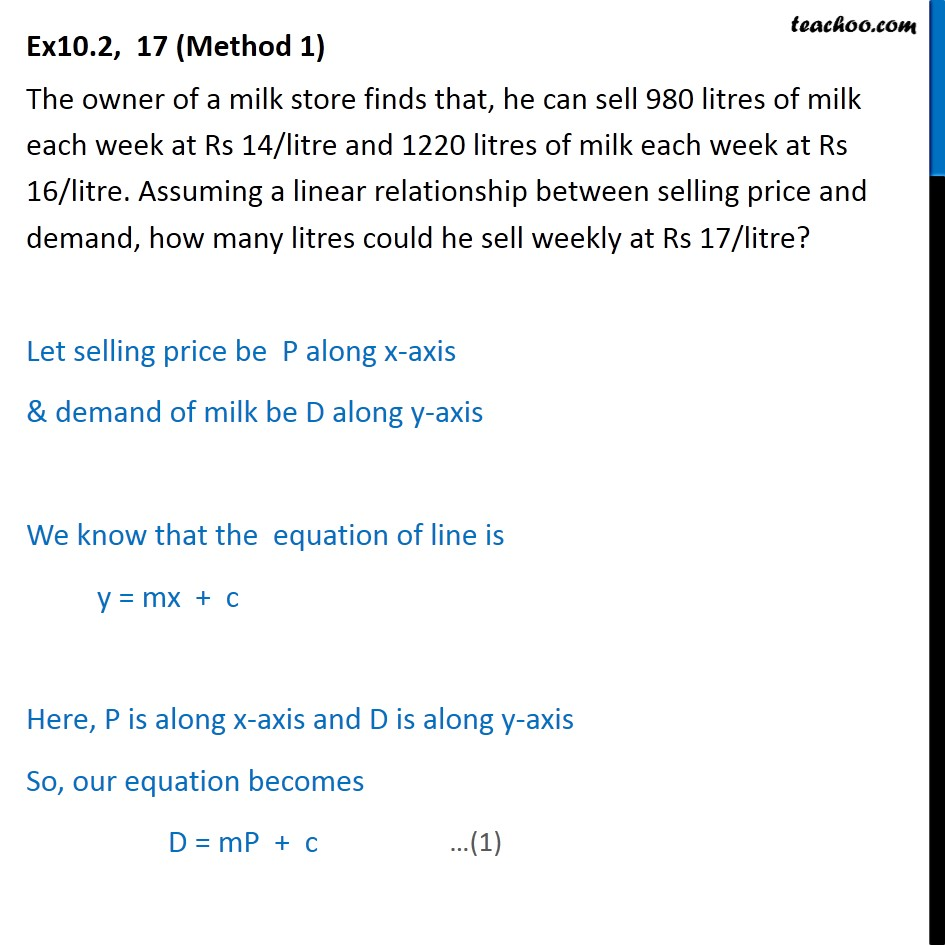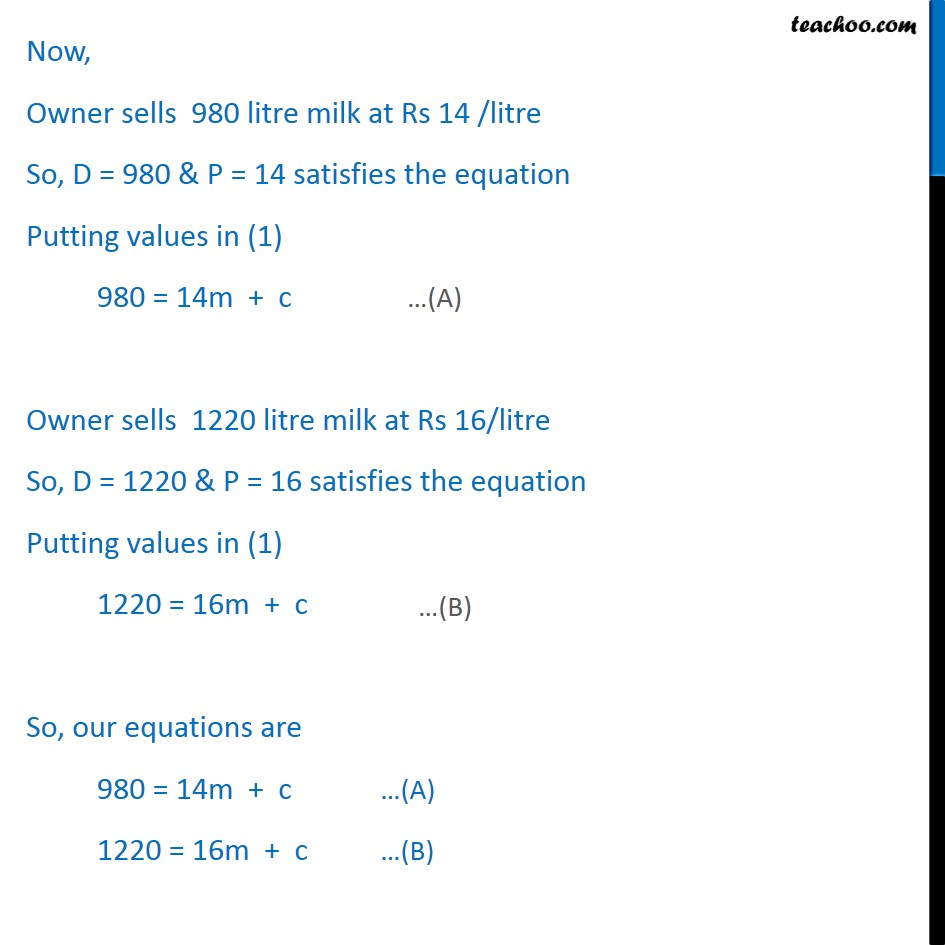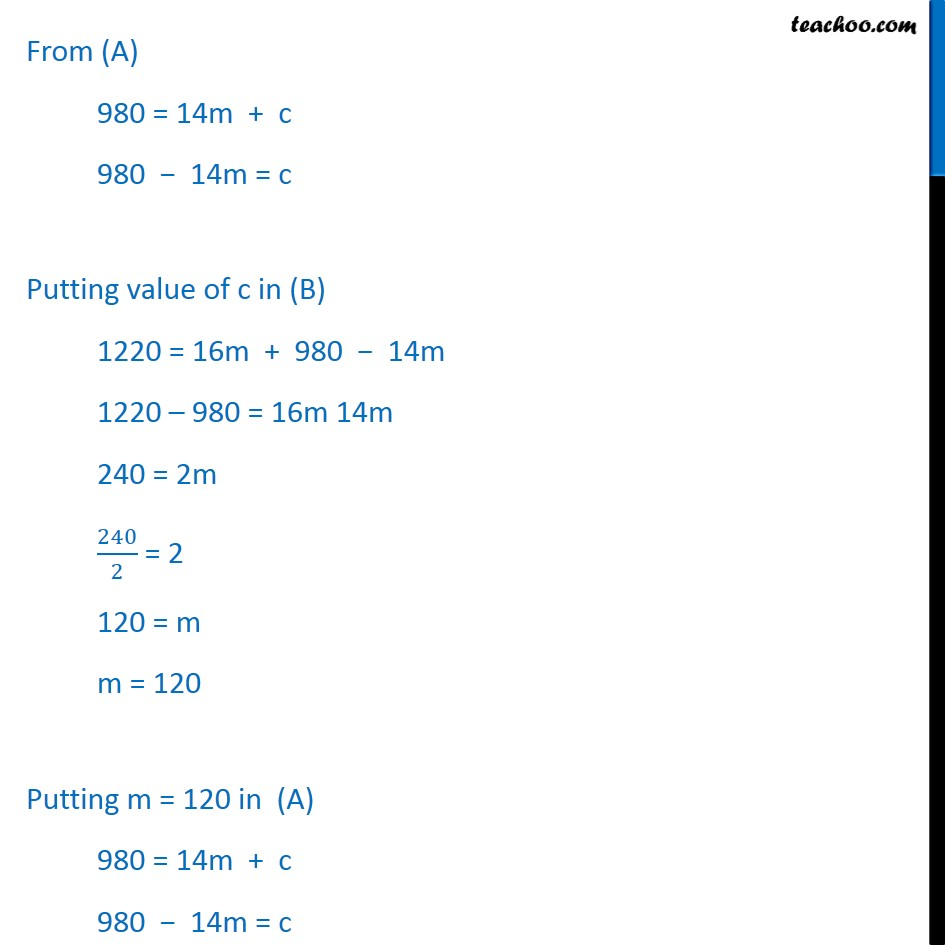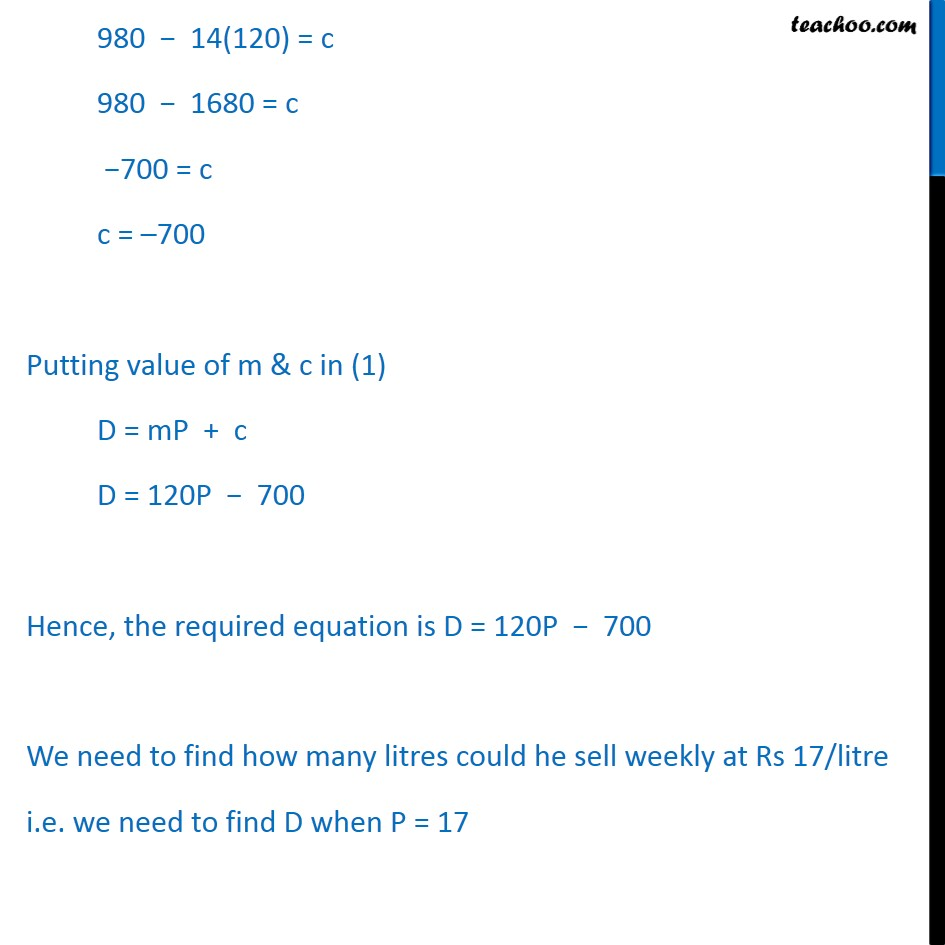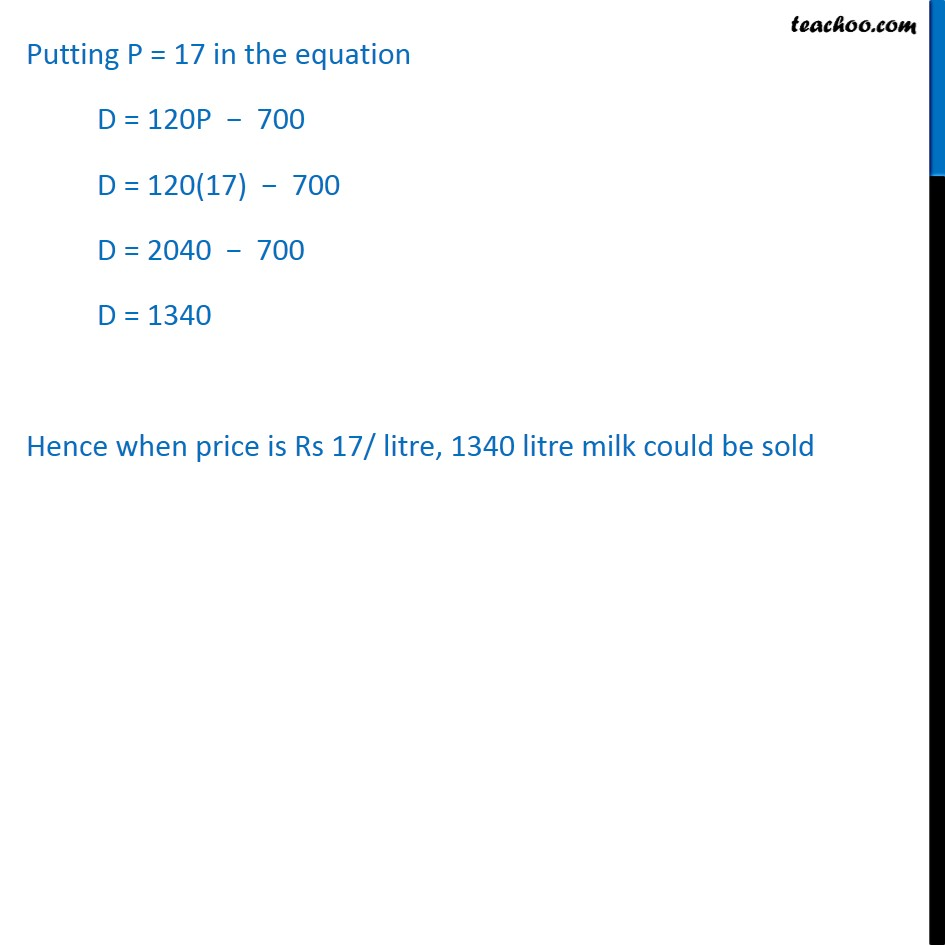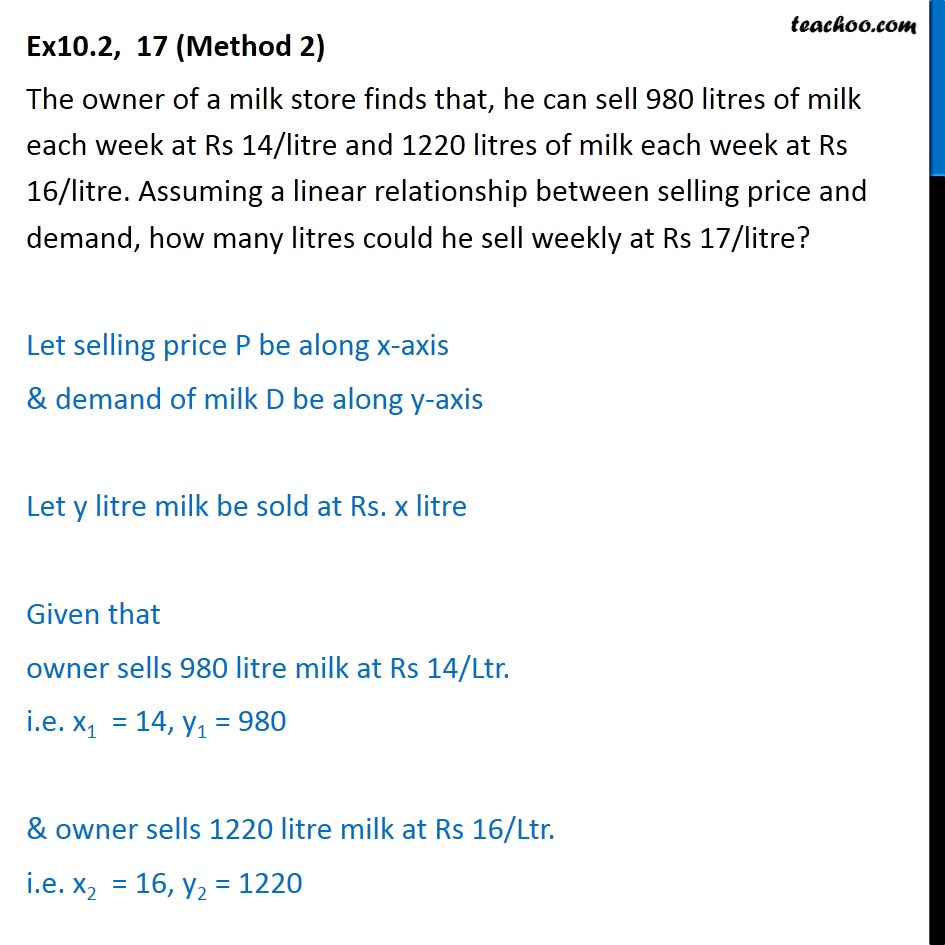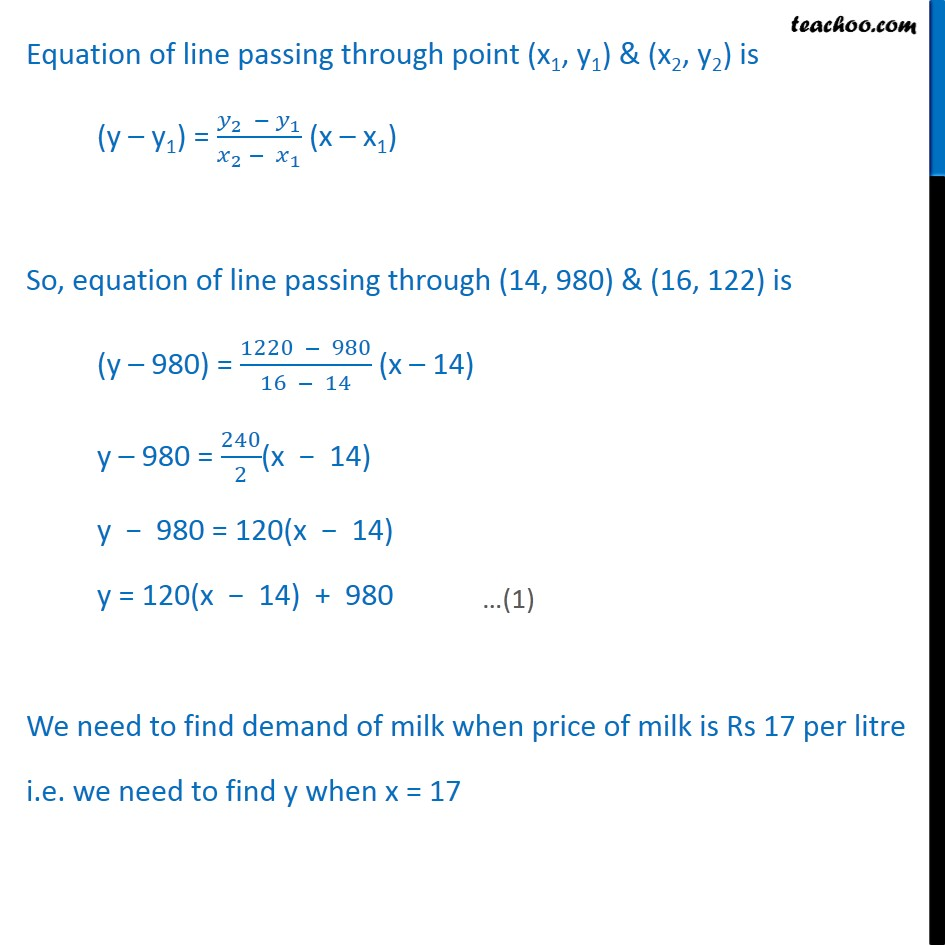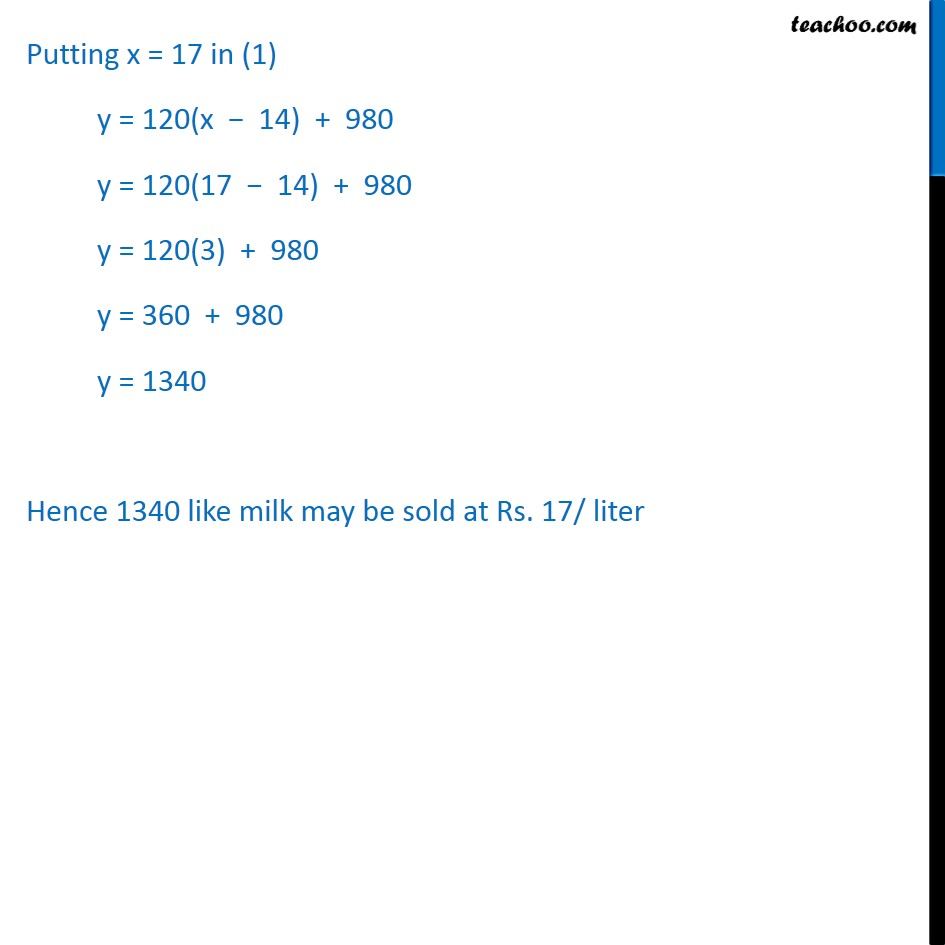1. Chapter 10 Class 11 Straight Lines
2. Serial order wise
3. Ex 10.2

Transcript

Ex10.2, 17 (Method 1) The owner of a milk store finds that, he can sell 980 litres of milk each week at Rs 14/litre and 1220 litres of milk each week at Rs 16/litre. Assuming a linear relationship between selling price and demand, how many litres could he sell weekly at Rs 17/litre? Let selling price be P along x-axis & demand of milk be D along y-axis We know that the equation of line is y = mx + c Here, P is along x-axis and D is along y-axis So, our equation becomes D = mP + c Now, Owner sells 980 litre milk at Rs 14 /litre So, D = 980 & P = 14 satisfies the equation Putting values in (1) 980 = 14m + c Owner sells 1220 litre milk at Rs 16/litre So, D = 1220 & P = 16 satisfies the equation Putting values in (1) 1220 = 16m + c So, our equations are 980 = 14m + c …(A) 1220 = 16m + c …(B) From (A) 980 = 14m + c 980 − 14m = c Putting value of c in (B) 1220 = 16m + 980 − 14m 1220 – 980 = 16m 14m 240 = 2m 240/2 = 2 120 = m m = 120 Putting m = 120 in (A) 980 = 14m + c 980 − 14m = c 980 − 14(120) = c 980 − 1680 = c −700 = c c = –700 Putting value of m & c in (1) D = mP + c D = 120P − 700 Hence, the required equation is D = 120P − 700 We need to find how many litres could he sell weekly at Rs 17/litre i.e. we need to find D when P = 17 Putting P = 17 in the equation D = 120P − 700 D = 120(17) − 700 D = 2040 − 700 D = 1340 Hence when price is Rs 17/ litre, 1340 litre milk could be sold Ex10.2, 17 (Method 2) The owner of a milk store finds that, he can sell 980 litres of milk each week at Rs 14/litre and 1220 litres of milk each week at Rs 16/litre. Assuming a linear relationship between selling price and demand, how many litres could he sell weekly at Rs 17/litre? Let selling price P be along x-axis & demand of milk D be along y-axis Let y litre milk be sold at Rs. x litre Given that owner sells 980 litre milk at Rs 14/Ltr. i.e. x1 = 14, y1 = 980 & owner sells 1220 litre milk at Rs 16/Ltr. i.e. x2 = 16, y2 = 1220 Equation of line passing through point (x1, y1) & (x2, y2) is (y – y1) = (𝑦_2 − 𝑦_1)/(𝑥_2 − 𝑥_1 ) (x – x1) So, equation of line passing through (14, 980) & (16, 122) is (y – 980) = (1220 − 980)/(16 − 14) (x – 14) y – 980 = 240/2(x − 14) y − 980 = 120(x − 14) y = 120(x − 14) + 980 We need to find demand of milk when price of milk is Rs 17 per litre i.e. we need to find y when x = 17 Putting x = 17 in (1) y = 120(x − 14) + 980 y = 120(17 − 14) + 980 y = 120(3) + 980 y = 360 + 980 y = 1340 Hence 1340 like milk may be sold at Rs. 17/ liter

Ex 10.2